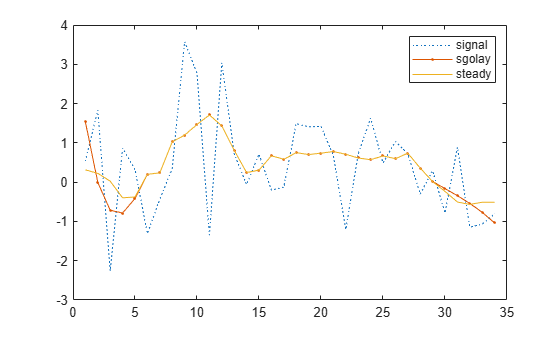sgolayfilt

Savitzky-Golay filtering

Description

example

y = sgolayfilt(x,order,framelen) applies a Savitzky-Golay finite impulse response (FIR) smoothing filter of polynomial order order and frame length framelen to the data in vector x. If x is a matrix, then sgolayfilt operates on each column.

example

y = sgolayfilt(x,order,framelen,weights) specifies a weighting vector to use during the least-squares minimization.

y = sgolayfilt(x,order,framelen,weights,dim) specifies the dimension along which the filter operates.

Examples

collapse all

Generate a random signal and smooth it using sgolayfilt. Specify a polynomial order of 3 and a frame length of 11. Plot the original and smoothed signals.

order = 3;
framelen = 11;

lx = 34;
x = randn(lx,1);

sgf = sgolayfilt(x,order,framelen);

plot(x,':')
hold on
plot(sgf,'.-')
legend('signal','sgolay')The sgolayfilt function performs most of the filtering by convolving the signal with the center row of B, the output of sgolay. The result is the steady-state portion of the filtered signal. Generate and plot this portion.

m = (framelen-1)/2;

B = sgolay(order,framelen);Samples close to the signal edges cannot be placed at the center of a symmetric window and have to be treated differently.

To determine the startup transient, matrix multiply the first (framelen-1)/2 rows of B by the first framelen samples of the signal.

ybeg = B(1:m,:)*x(1:framelen);

To determine the terminal transient, matrix multiply the final (framelen-1)/2 rows of B by the final framelen samples of the signal.

yend = B(framelen-m+1:framelen,:)*x(lx-framelen+1:lx);

Concatenate the transients and the steady-state portion to generate the complete signal.

cmplt(1:m) = ybeg;
cmplt(lx-m+1:lx) = yend;

plot(cmplt)
hold offAdding weights to the minimization breaks the symmetry of B and requires extra steps for a proper solution.

Load a speech signal sampled at ${\mathit{F}}_{\mathit{s}}=7418\text{\hspace{0.17em}}\mathrm{Hz}$. The file contains a recording of a female voice saying the word "MATLAB®."

t = (0:length(mtlb)-1)/Fs;

Smooth the signal by applying a Savitzky-Golay filter of polynomial order 9 to data frames of length 21. Plot the original and filtered signals. Zoom in on a 0.02-second interval.

rd = 9;
fl = 21;

smtlb = sgolayfilt(mtlb,rd,fl);

subplot(2,1,1)
plot(t,mtlb)
axis([0.2 0.22 -3 2])
title('Original')
grid

subplot(2,1,2)
plot(t,smtlb)
axis([0.2 0.22 -3 2])
title('Filtered')
gridRepeat the calculation, but now use a Kaiser window as a weighting vector. Specify a shape factor $\beta =38$. Plot the new filtered signal.

kmtlb = sgolayfilt(mtlb,rd,fl,kaiser(fl,38));

subplot(2,1,2)
hold on
plot(t,kmtlb)
axis([0.2 0.22 -3 2])
hold offInput Arguments

collapse all

Input signal, specified as a vector or matrix.

Data Types: single | double

Polynomial order, specified as a positive integer. order must be smaller than framelen. If order = framelen – 1, the filter produces no smoothing.

Data Types: single | double

Frame length, specified as a positive odd integer.

Data Types: single | double

Weighting array, specified as a real positive vector or matrix of length framelen.

Data Types: single | double

Dimension to filter along, specified as a positive integer scalar. By default, sgolayfilt operates along the first dimension of x whose size is greater than 1.

Data Types: single | double

Output Arguments

collapse all

Filtered signal, returned as a vector or matrix.

Tips

Savitzky-Golay smoothing filters are typically used to "smooth out" a noisy signal whose frequency span (without noise) is large. They are also called digital smoothing polynomial filters or least-squares smoothing filters. Savitzky-Golay filters perform better in some applications than standard averaging FIR filters, which tend to filter high-frequency content along with the noise. Savitzky-Golay filters are more effective at preserving high frequency signal components but less successful at rejecting noise.

Savitzky-Golay filters are optimal in the sense that they minimize the least-squares error in fitting a polynomial to frames of noisy data. See sgolay for more information about the Savitzky-Golay algorithm.

 Orfanidis, Sophocles J. Introduction to Signal Processing. Englewood Cliffs, NJ: Prentice Hall, 1996.

 Schafer, Ronald. “What Is a Savitzky-Golay Filter? [Lecture Notes].” IEEE Signal Processing Magazine 28, no. 4 (July 2011): 111–17. https://doi.org/10.1109/MSP.2011.941097.